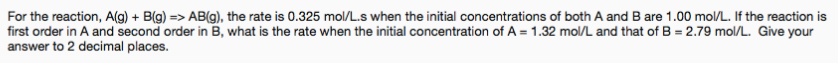# For the reaction, A(g) + B(g) => AB(g), the rate is 0.325 mol/L.s when the initial concentrations of both A and B are 1.00 mol/L. If the reaction is first order in A and second order in B, what is the rate when the initial concentration of A = 1.32 mol/L and that of B = 2.79 mol/L. Give your answer to 2 decimal places.